# A Stone connected to chain is thrown vertically

Rikudo
Homework Statement:
see attachment
Relevant Equations:
impulse-momentum, newton's 2nd law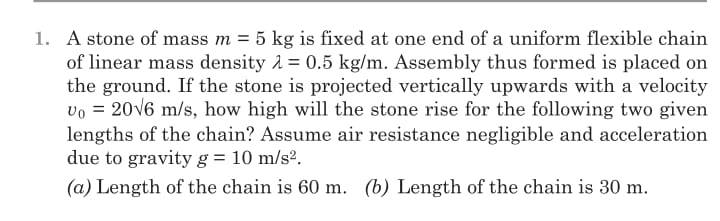In order to be able to solve the problem, I think I must find the equation of ##h## with respect to ##\dot h##.
Assuming that ##F## is the action-reaction force between the stone and the end of chain, then the Newton's equation
For the stone:$$-F-mg=m \ddot h$$ $$-\int (F+mg)dh = \frac 1 2 m(\dot h^2 - Vo^2)...(1)$$

For the chain in the air:
a. Equation for the momentum: $$p=\lambda h \dot h...(2)$$ b. equation for impulse-momentum: $$\frac {dh}{dh} \frac {dp}{dt} = F-\lambda gh$$ $$\int \dot h dp = \int Fdh - \lambda gh^2/2...(3)$$
Substituting the value of ##\int F dh## from (1) into (3) will give us $$\int \dot h dp = -mgh -\frac 1 2 m(\dot h^2 - Vo^2) - \lambda gh^2/2...(4)$$
taking the derivative of p, then substitute it into (4) :
$$\int \dot h (\lambda \dot h dh+ \lambda h d \dot h) = -mgh -\frac 1 2 m(\dot h^2 - Vo^2) - \lambda gh^2/2$$

After that, what should I do next?

•Delta2

Gold Member
I think you need to take a few steps back and write the free body diagram of the stone? What are the forces acting on it at some time ## t > 0 ## ? Maybe you are trying to do something fancy that I don't understand with Lagrangian Mechanics, but this seems to me like it should be solving a Second Order Linear Non Homogeneous ODE using Newtons Second Law?

Last edited:
Rikudo
Sorry, but my answer is still the same as the one in (1); mg and F, and their directions are downward.

Gold Member
Sorry, but my answer is still the same as the one in (1); mg and F, and their directions are downward.
Yeah, but ##F## is simply the force of weight of the chain acting on the stone. hint: It's a simple function of ##h##.

Rikudo
I don't think so.
F also includes the force needed to make a small part of the chain on the floor (we can call it ##dm##) start moving

Homework Helper
Gold Member
I don't think so.
F also includes the force needed to make a small part of the chain on the floor (we can call it ##dm##) start moving
I agree. However, I think it would be simpler to write a single equation of motion for the mass plus the part of the chain that's in the air.

Homework Helper
Gold Member
2022 Award
Chain/rope problems like this are tricky, even generating arguments between experts. E.g. search for "chain fountain". A key question is what exactly happens as successive links rise from the ground?
The first difficulty is that we are not told how the assembly is laid out. Is the chain in a horizontal line or in a pile of negligible size? The first option leads to horizontal forces in the chain, so let's delete that one.

One approach is to assume work is conserved, but is that justified?
Another is to use momentum. In this approach we could assume each lifted link is instantaneously accelerated from rest to the current speed of the rising chain.
Worryingly, these two approaches will yield different answers. The momentum analysis says that half the work done in lifting the links off the ground is lost.

In reality, the answer tends to lie between the two. Yes, there is some work lost, but the momentum argument overlooks ways in which apparently lost work can be recovered. E.g. lifting a link off the ground may involve rotating it, so some work goes into rotational energy; but at a later point, that rotational energy may be partly used in lifting the next link.

But if we suppose all the links stand erect initially then there is no rotation. In this case, there is a collision each time a link rises to the point where it engages the upper end of the next link. If the collision is inelastic then clearly a lot of work is lost, but even if we take it to be elastic a lot of the work may end up as internal oscillations of the chain, not necessarily contributing to raising successive links. Perhaps there turns out to be a most efficient speed for lifting the chain that depends on the link length. Dimensional analysis suggests it would be some constant multiple of ##\sqrt{gl_{link}}##.

Last edited:
•collinsmark, Delta2 and Lnewqban
Rikudo
Ah. I just noticed that I haven't tell you that the system for the momentum equation (equation 2 ) is : small part of the chain on floor + mid-air chain.

Rikudo
... but this seems to me like it should be solving a Second Order Linear Non Homogeneous ODE using Newtons Second Law?
Well, when I tried a slightly different method, I got an equation of 2nd ODE.
The result is cumbersome,though.

$$-F-mg=m \ddot h...(1)$$ Using impulse-momentum:
$$\frac {dp}{dt} = F-\lambda gh$$ $$\lambda (\dot h^2 + h \ddot h) = F-\lambda gh$$
Substituting the value of F from the equation above into (1) gives us:
$$-\lambda \dot h^2 -\lambda h \ddot h - \lambda gh -mg = m \ddot h$$

I wonder whether there are any other methods in solving the problem...

Homework Helper
Gold Member
2022 Award
I wonder whether there are any other methods in solving the problem...
What do you get if you just assume work is conserved?
Using the momentum method I referred to in post #7 I get the equation ##u\ddot u+\dot u^2=-\lambda gu##, where ##u=m+\lambda h##. This has no solution in a standard closed form.

Last edited:
Gold Member
I had no idea this problem was that far over my head (to be fair you put it in "Introductory Physics" ).

I simply thought the chain was in a " idealized pile" on the floor, and the links are effectively "at rest" until they climb out.

$$m \ddot{h} = - ( m + \lambda h )g$$

But I'm looking in my differential equation textbook and I see that even that must be incorrect...I admittedly don't see why?

Anyhow, here is a similar problem, and it was answered in the back of the book.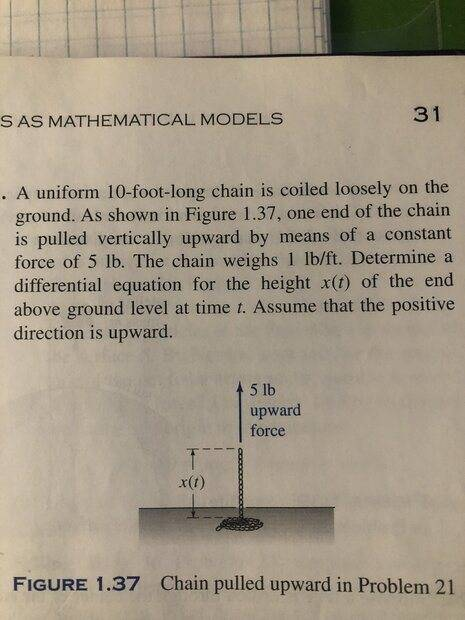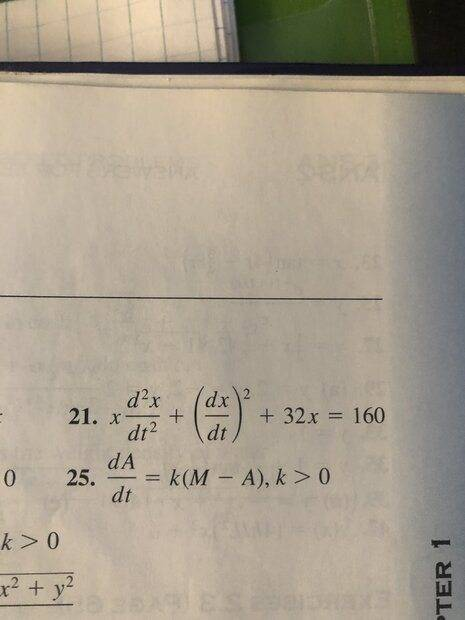•Delta2
Homework Helper
Gold Member
2022 Award
I had no idea this problem was that far over my head (to be fair you put it in "Introductory Physics" ).

I simply thought the chain was in a " idealized pile" on the floor, and the links are effectively "at rest" until they climb out.

$$m \ddot{h} = - ( m + \lambda h )g$$

But I'm looking in my differential equation textbook and I see that even that must be incorrect...I admittedly don't see why?

Anyhow, here is a similar problem, and it was answered in the back of the book.

View attachment 300817
View attachment 300818
That concurs with the momentum solution I discussed above. But as I wrote, there are reasons why this may exaggerate the work lost.

•Delta2
Gold Member
That concurs with the momentum solution I discussed above. But as I wrote, there are reasons why this may exaggerate the work lost.
I'm assuming you are talking about the "similar problem" I posted, and not what I thought was the governing equation for the chain attached to a stone.

So where does the ## \left( \frac{dx}{dt} \right)^2## come from in the FBD for the problem I show in the picture? I'm assuming it comes from the chain in the pile moving, but I don't know why that "must" to move for this to be a reasonable representation?

Last edited:
Homework Helper
Gold Member
Using the momentum method I referred to in post #7 I get the equation ##u\ddot u+\dot u^2=-\lambda gu##, where ##u=m+\lambda h##. This has no solution in a standard closed form.
##u\ddot u+\dot u^2=-\lambda gu##
This equation can be solved analytically perhaps not to find ##u(t)## but an expression that can be used to answer the question posed by the problem.
In the interests of transparency, rename
##u =M=m+\lambda h## (the mass of the stone + chain system that is in free fall)
##v=\dot h## (the speed of the stone + chain system that is in free fall)
Then
##\ddot u=\lambda \dot v##
##\dot u^2=\lambda^2 v^2=\lambda v\dot M##
The equation becomes
##\lambda M \dot v+\lambda v\dot M=-\lambda M g##
and with ##P=Mv## simplifies to
##\dfrac{dP}{dt}=-Mg##.
To solve it, consider that ##\dfrac{dP}{dt}=\dfrac{dP}{dh}\dfrac{dh}{dt}=\dfrac{P}{M}\dfrac{dP}{dh}##
so that, finally, ##\dfrac{P}{M}\dfrac{dP}{dh}=-Mg##.
When ##M=m+\lambda h## is put back into this equation, it separates and one can find ##P(h)## . Then one has to solve the equation ##P(h_{max})=0## for the maximum height.

When I did all that, I found a maximum height that is less than 30 m which makes me suspicious because the answer to both parts (a) and (b) would be the same. Also the initial speed of ##20\sqrt{6}## m/s is most likely intended to give a simple answer which I did not get.

We can subdivide the fixed-mass system consisting of the entire length of chain plus stone into two subsystems, ##S_1## in free fall and ##S_2## lying on the table. Then ##\dfrac{dP_1}{dt}=-Mg+F_{12}## and ##\dfrac{dP_2}{dt}=F_{21}=-F_{12}## so that for the whole system $$\frac{dP}{dt}=\frac{d}{dt}(P_1+P_2)=\frac{dP_1}{dt}+\frac{dP_2}{dt}=-Mg.$$

Last edited:
•haruspex and Lnewqban
Homework Helper
Gold Member
2022 Award
I found a maximum height that is less than 30 m which makes me suspicious because the answer to both parts (a) and (b) would be the same. Also the initial speed of 206 m/s is most likely intended to give a simple answer which I did not get.
Which reinforces my suspicion that the author took work to be conserved. While that is not entirely unjustified, it needs to be stated in the problem.

Gold Member
After some head scratching, I think I'm finally catching on: Since this is supposed to be Introductory Physics, I'll fill in the missing details for other noobs like me:

$$p(t + \Delta t ) - p(t) = F_{net} \Delta t$$

$$m( v + \Delta v) + \lambda ( h + \Delta h )( v + \Delta v ) - ( mv + \lambda h v) = F_{net} \Delta t$$

$$F_{net} = - ( mg + \lambda h g )$$

Then after expansion, and neglecting higher order differentials ## \Delta h \Delta v ##:

$$m \Delta v + \lambda \Delta h v + \lambda h \Delta v = - ( mg + \lambda h g ) \Delta t$$

Furthermore, dividing through by ##\Delta t## and taking the limit as ##t \to 0## and substituting ## v = \dot{h} ##:

$$m \ddot{h} + \lambda { \dot{h} }^2 + \lambda h \ddot{h} = -mg - \lambda h g$$

and that is the same EOM that the OP has determined. Which is apparently neither wrong, nor right, but may in fact not be what the question designer was looking for...Last edited:
•Delta2
Homework Helper
Gold Member
Which reinforces my suspicion that the author took work to be conserved. While that is not entirely unjustified, it needs to be stated in the problem.
It looks like the author had mechanical energy conservation in mind. Under that assumption
$$\frac{1}{2}mv_0^2=mgh_{\text{max}}+(\lambda h_{\text{max}}) g\frac{h_{\text{max}}}{2}.$$The acceptable root of the quadratic is $$h_{\text{max}}=\frac{1}{\lambda g}\left[{\sqrt{mg(mg+\lambda v_0^2)}-mg}\right]=40 ~\text{m}$$ Under this assumption, when the length of the chain is 60 m, the maximum height is 40 m but when it is 30 m, it's more than that - I won't bother calculating how much more. I think this settles what the author had in mind and uses math at the level of an introductory physics problem.

•Lnewqban
Rikudo
It looks like the author had mechanical energy conservation in mind. Under that assumption
$$\frac{1}{2}mv_0^2=mgh_{\text{max}}+(\lambda h_{\text{max}}) g\frac{h_{\text{max}}}{2}.$$
At the beginning of motion, why is there no KE for ##dm## part of the chain?
so: $$KE_{initial} = \frac{1}{2}(m+\lambda dh)v_0^2$$
Is there any reason why we can neglect it?

Homework Helper
Gold Member
2022 Award
Is there any reason why we can neglect it?
Yes, dh is the only infinitesimal in that equation. In the limit, it vanishes.

Gold Member
It looks like the author had mechanical energy conservation in mind. Under that assumption
$$\frac{1}{2}mv_0^2=mgh_{\text{max}}+(\lambda h_{\text{max}}) g\frac{h_{\text{max}}}{2}.$$The acceptable root of the quadratic is $$h_{\text{max}}=\frac{1}{\lambda g}\left[{\sqrt{mg(mg+\lambda v_0^2)}-mg}\right]=40 ~\text{m}$$ Under this assumption, when the length of the chain is 60 m, the maximum height is 40 m but when it is 30 m, it's more than that - I won't bother calculating how much more. I think this settles what the author had in mind and uses math at the level of an introductory physics problem.
"Under this assumption, when the length of the chain is 60 m, the maximum height is 40 m but when it is 30 m, it's more than that"

Something isn't right, because 20 m of chain is still in a pile on the ground?

The question is how high the stone rises.

So if we let ## h ## be the CM of the chain and ## l ## be its length:

$$\frac{1}{2} m v_o^2 = mg \left ( h + \frac{l}{2} \right) + \lambda l g \frac{h}{2}$$

Then for the stone ## h_{max}##is given by:

$$h_{max} = h + \frac{l}{2}$$

?

Last edited:
•Delta2
Homework Helper
Gold Member
2022 Award
Something isn't right, because 20 m of chain is still in a pile on the ground?
Why is that a problem?
The energy in the stone is insufficient to lift itself and an attached chain more than 40m. If a 60m chain, 20m remain on the ground.
If the chain is less than 40m then the stone does less work in lifting it 40m, so can go higher.

•erobz
Gold Member
Why is that a problem?
The energy in the stone is insufficient to lift itself and an attached chain more than 40m. If a 60m chain, 20m remain on the ground.
If the chain is less than 40m then the stone does less work in lifting it 40m, so can go higher.
How is the weight of the chain lying on the ground contributing to the mass in the potential energy on the RHS?

Last edited:
Rikudo
So if we let ## h ## be the CM of the chain and ## l ## be its length:
Do you mean: ##l## is the length of the chain in the air?
Then for the stone ## h_{max}##is given by:

$$h_{max} = h + \frac{l}{2}$$

?
Yes, but this is only if the chain length ≥ 40m. Also, this formula works at any height of the stone (not only when it is at maximum height).
(note that here, I assume ##l## is the length of mid-air-chain)

Homework Helper
Gold Member
It isn’t. The total length of the chain does not figure in the calculation. Only the length above the table does.

•erobz
Rikudo
One approach is to assume work is conserved, but is that justified?
Another is to use momentum. In this approach we could assume each lifted link is instantaneously accelerated from rest to the current speed of the rising chain.
If we assume that energy is conserved, then how different will the way for a link at rest is accelerated? (Especially in this problem's case)

Gold Member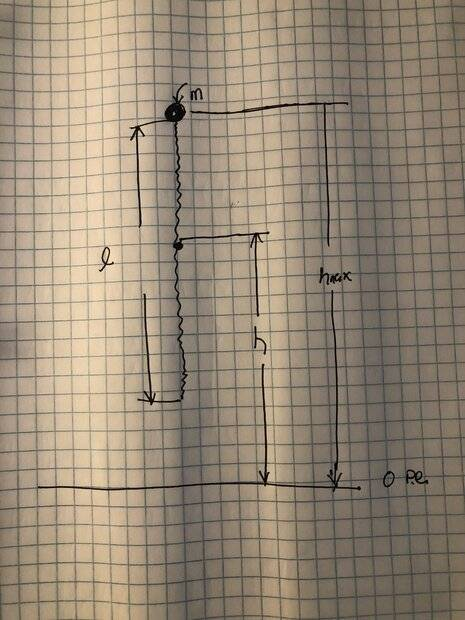This is what I was thinking, what is wrong? The fact that it assumes the chain fully lifts off the ground?

$$\frac{1}{2} m v_o^2 = mg \left ( h + \frac{l}{2} \right) + \lambda l g \frac{h}{2}$$

$$h_{max} = h + \frac{l}{2}$$

Homework Helper
Gold Member
View attachment 300873

This is what I was thinking, what is wrong? The fact that it assumes the chain fully lifts off the ground?

$$\frac{1}{2} m v_o^2 = mg \left ( h + \frac{l}{2} \right) + \lambda l g \frac{h}{2}$$

$$h_{max} = h + \frac{l}{2}$$
Are you trying to find how high the 30 m chain is lifted off the ground? If so, you first need to find how fast it is moving when the its end has been just lifted off.

Rikudo
The height of the stone must be higher than the height of the CM of the chain?
Yes.

Also, about ##h_{max} = h + \frac{l}{2}##.
When I wrote post #23, I thought ##h## is measured from the end of the chain.

So, If ##h## is measured from the table, then the formula works with no limitation of total chain length.

Gold Member
Ok...I see it now. Thanks for the clarification. I shouldn't do this before a cup of coffee (maybe not at all...but I do like it! ). I imagine it goes without saying, but I don't have a Physics degree...go easy on me ( I don't want to reveal what degree I do have to protect its integrity..haha ).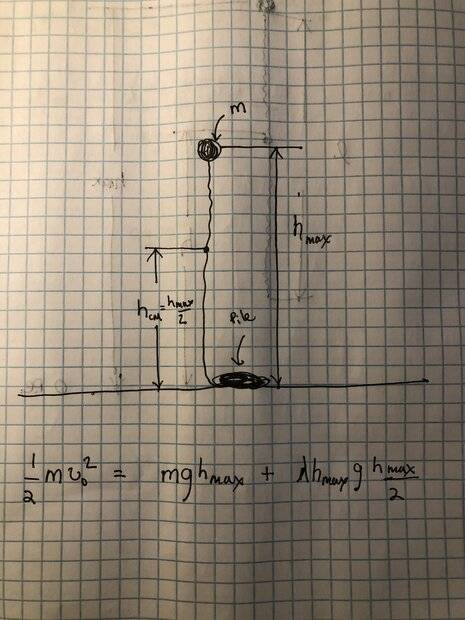Sorry for annoying you all...Homework Helper
Gold Member
2022 Award
If we assume that energy is conserved, then how different will the way for a link at rest is accelerated? (Especially in this problem's case)
As I wrote in post #7:
lifting a link off the ground may involve rotating it, so some work goes into rotational energy; but at a later point, that rotational energy may be partly used in lifting the next link.
Consider the last link lifted, link 1, erect but still touching the table. If the next link, link 2, lies flat, they form an L shape. As link 2 is lifted, from one end, it rotates. When it reaches vertical it still has rotational inertia. No mechanical work has been lost at this point; the missing energy (according to the linear momentum analysis) is in that rotation.
As link 2 passes vertical, rotating clockwise say, its lower end starts to lift link 3. That puts an anticlockwise torque on link 2, so link 2's rotation slows as some of that rotational energy goes into lifting link 3.
Most likely, not all the rotational energy gets recovered. Some may remain as oscillations that soon die away. Moreover, the situation at the start of lifting one link need not be identical to that at the start of lifting the next. This makes it very difficult to estimate what fraction of work is lost.
Indeed, it may well be that chaotic behaviour can arise. By dimensional analysis, for constant speed the critical parameter will be ##\frac{v^2}{gL}##, where L is the link length and v the lifting speed.

Last edited:
•Lnewqban
••